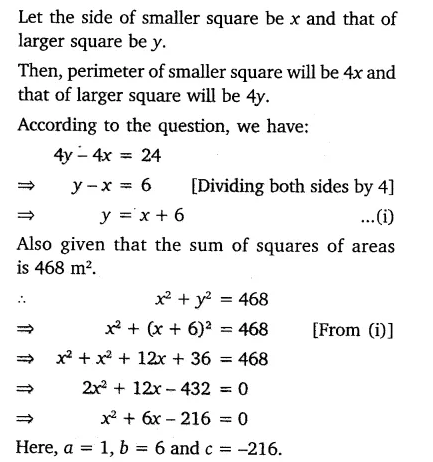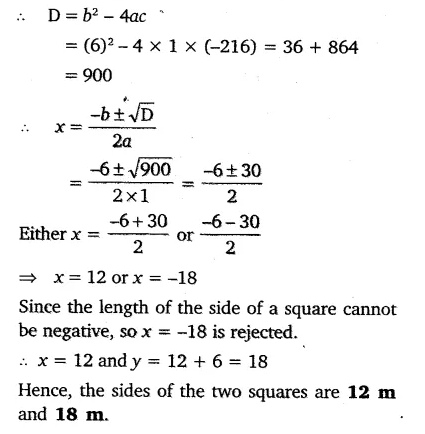# Sum of the areas of two squares is 468 m2. If the difference of their perimeters is 24 m,

Sum of the areas of two squares is 468 m2. If the difference of their perimeters is 24 m, find the sides of the two squares.